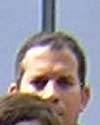#Wolfram Summer School

# Alumni# Johan Veerman

Summer School

Class of 2004

## Bio

Johan was born in Lima, Perú. He did undergraduate studies in business administration and graduate studies in physics. Along with two other colleagues from the physics section at Pontificia Universidad Católica del Perú, he has formed a Complex Systems Research Group where they plan to focus on NKS. Johan is interested in a wide variety of subjects, ranging from cosmology to myrmecology. When not trying to figure out how the universe works he likes to go scuba diving, play soccer, or just read a good novel.

## Project: Calculating Mathematical Functions with Cellular Automata

There are four things we know about cellular automata:

1. They are capable of universal computation.
2. They can emulate other systems.
3. They can calculate mathematical functions.
4. We don’t know yet the mechanisms behind how they can do these calculations.

If we could find an algebra or an axiom system based on CA then it would be possible to build specific rules to evaluate a wide variety of mathematical problems. This is the long-term goal of this project. In the short term, finding some CA rules that perform mathematical functions is the first step in extracting the mechanism that lies inside the calculations. We used two different approaches:

• Conducting searches to find rules that comply with given calculation requirements
• Constructing specific rules that perform certain calculations

When we take a few examples of CA that calculate mathematical functions, we find that there are always one or more particles present, moving, bouncing around, that carry the information from one place on the grid to another and that perform the computations.

We have shown an example of a CA rule that does addition given an input in binary. Rules for calculating in other bases will help to find the mechanisms behind the computations.